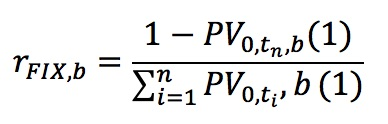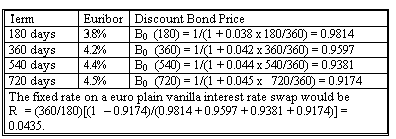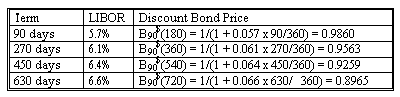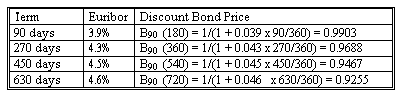### Seeing is believing!

Before you order, simply sign up for a free user account and in seconds you'll be experiencing the best in CFA exam preparation.

##### Subject 9. Currency Swap Contracts
There are four types of currency swaps:

• Pay one currency fixed, receive the other fixed.
• Pay one currency fixed, receive the other floating.
• Pay one currency floating, receive the other fixed.
• Pay one currency floating, receive the other floating.

A few key features:

• They often (but not always) involve the exchange of notional amounts.
• Payments are not netted, as they are in different currencies.
• Each leg of the swap can be either fixed or floating.

Note the relationship between interest rate and currency swaps (c1: currency 1; c2: currency 2).Pricing a Currency Swap

Obviously, for the last type of currency swap, in which both sides pay floating, we do not need to price the swap because both sides pay a floating rate.

Take fixed-for-fixed currency swaps as an example. Currency swap pricing has three key variables: two fixed interest rates and one notional amount. The present value of the payments made should be equal to the present value of the payments received.

We price currency swaps in the same way we learned to price interest rate swaps.Example

Reston Technology enters into currency swap with GSI. Reston will pay euros based on NP of €10 million semi-annually for two years. GSI will pay dollars semi-annually for two years. Notional principals will be exchanged. The spot exchange rate is \$0.9804/€ at swap initiation time.

The following term structures, discount bond prices, and the resulting swap fixed rates are given below:• Euro notional principal is NP = €10 million. With S0 = \$0.9804, the US\$ notional principal is NP\$ = 1 * S0 = \$9,804,000.
• The US\$ interest rate is 6.1%.
• The € interest rate is 4.35%.

As you can see, we only need to deal with the spot exchange rate. We don't need to address future foreign currency transactions.

Valuing a Currency Swap

During the life of the swap, we value it by finding the difference in the present values of the two streams of payments, adjusting for the notional principals, and converting to a common currency. Assume the new exchange rate is \$0.9790 three months later.

Three months into the Reston-GSI swap, the new term structures and zero coupon bond prices for dollars are:The present value of the dollar fixed payments of 0.061 (180/360) plus a \$1 notional principal is: 0.061 (180/360) (0.9860 + 0.9563 + 0.9259 + 0.8965) + 1 (0.8965) = 1.01132335.

The new term structure and discount bond prices for the euro are:The present value of the euro fixed payments of 0.0435 (180/360) plus a €1 notional principal is: 0.0435 (180/360) (0.9903 + 0.9688 + 0.9467 + 0.9255) + 1 (0.9255) = 1.00883078.

The value of the swap:

• Dollars fixed for NP of \$9.804 million = \$9,804,000(1.01132335) = \$9,915,014
• Euros fixed for NP of €10 million = €10,000,000(1.00883078) = €10,088,308
• Value of swap to pay € fixed, receive \$ fixed: \$9,915,014 - €10,088,308(\$0.9790/€) = \$38,560

If the swap had been designed with floating payments, the present value of the dollar floating payments would be found by discounting the next floating payment, which is at the original 180-day floating rate of 5.5%, plus the market value of the floating-rate bond on the next payment day: [1 + 0.055 (180/360)] 0.9860 = 1.013115.

If the payments were floating, the present value of the euro floating payments would be found by discounting the next floating payment, which is at the original 180-day floating rate of 3.8%, plus the market value of the floating-rate bond on the next payment date: [1.0 + 0.038 (180/360)] 0.9903 = 1.0091157.

User Comment
anamdi time consuming and confusing...
aravinda Actually this is same as the interest rate swap from the last reading...Here the exchange rate comes into picture ONLY in the end..when you calculate the value of Swap (received - payed amount)...
rhardin Check out the Schweser notes on this LOS... they were a little bit clear and simplified. But come back to AN for everything else!
Gooner7 finally working on a rates desk has some benefit on the exam!!
Tlhogi When working out the fixed rate at time 0, you use the 'hypothetical' notional principal of 1 for both currencies, with the value of the swap at zero.

But the actual notional principal is different to one i.e 1/S0, for the foreign currency. So when working out the present values of the foreign fixed and floating payments, you need to convert them using the actual notional principal expressed in terms of the foreign currency. Multiply by the notional principal of 1/S0.

Then to be able to value the currency swap, you convert these values into the home currency so that the value is calculated in one currency.
johntan1979 Totally agree that these are tough material... and these are only scratching the surface of the world of swaps.
Shaan23 Text is actually a good read for this....makes it simple